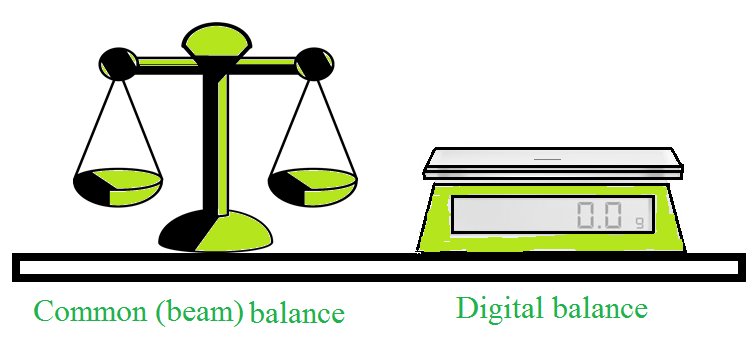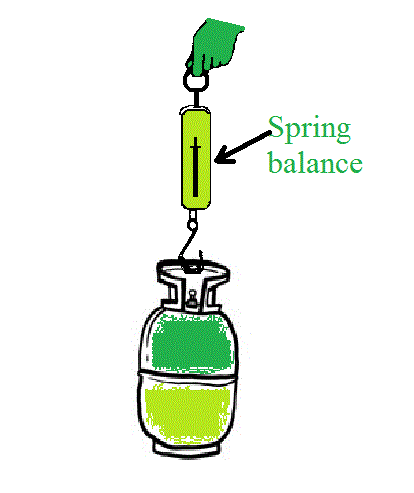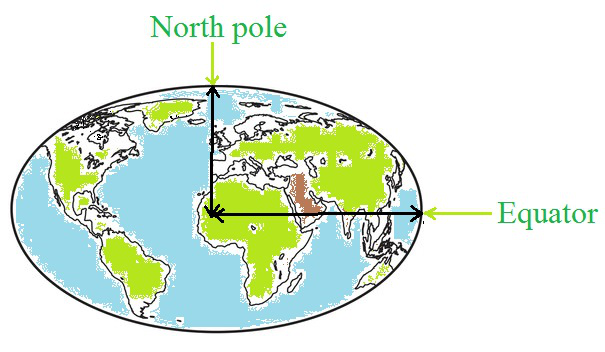# Mass and Weight

• Last Updated : 06 Jun, 2021

Weight is a measure of force, whereas mass is a measure of inertia. Many people use these two words interchangeably. This, however, is not the case. We can assist you in researching the numerous distinctions between mass and weight.

To change the speed or direction of an object, a force is required. If you drop anything from a great height, it will fall towards the earth’s surface. The planet revolves around the Sun and the moon revolves around the earth. There must be some force operating on objects, planets, and other celestial bodies. Isaac Newton noted that the same force is responsible for all these. This force is called the gravitational force.

Attention reader! All those who say programming isn't for kids, just haven't met the right mentors yet. Join the  Demo Class for First Step to Coding Course, specifically designed for students of class 8 to 12.

The students will get to learn more about the world of programming in these free classes which will definitely help them in making a wise career choice in the future.

### Mass

Mass is defined as the measure of the amount of matter present in a body. The mass of the body remains the same everywhere, irrespective of location. Thus, mass refers to both, the property of a physical body and the measure of the resistance of the object to acceleration when we apply a net force.

The inertia of an object is measured by its mass i.e. the mass of an object is the measure of its inertia. When the mass of the body increases there will be an increment in the inertia also.

The mass of the body does not change with the place. Whether an object is on the earth, the moon, or even in space, its mass remains constant. As a result, an object’s mass is constant and does not vary with its location.

The formula to calculate the mass of an object is:

Mass (m) = Volume (V) × Density (ρ)

or

m = V × ρ

It is a scalar quantity and its SI unit is kilogram (kg).

The mass of an object measured by using an ordinary balance, beam balance, or digital balance.An instrument that measures the mass of an object.

### Weight

The weight of an object is defined as the measure of the amount of force that acts on mass because of the pull of gravity. Weigh is the measure of force. Therefore, Weight will vary depending on the location.

The earth attracts every item with a force that is proportional to the object’s mass (m) and the acceleration due to gravity (g). The force that pulls an object towards the earth is known as its weight.

It is a vector quantity. It’s got the magnitude and directs toward the Earth’s center or other gravity.

The SI unit of weight is Newton, which is the same as the SI unit of force (N).

Weight of an object measured by measured using a spring balance.Acceleration due to gravity (g): Whenever an object falls towards the earth, it experiences acceleration. The earth’s gravitational force is responsible for this acceleration. This acceleration is known as the acceleration due to gravity. It is denoted by g. The unit of g is m s–2, which is the same as the unit of acceleration.

### Formula for Weight

Everything is attracted to the earth with a certain force and this force depends on the mass (m) of the object and the acceleration due to the gravity (g).

The Force can be expressed as,

F = m × a

where, m is the mass of an object and a is the acceleration of the body.

i.e.

F = m × g

The weight of an object is defined as the earth’s force of attraction on it and it is represented by W.

So the expression can be written as,

W = m × g

The weight is a downward-acting force that has both magnitude and direction so, it is a vector quantity.The distance between a point at the equator and the center of the earth and the distance between the point at the North Pole and the center of the earth.

The earth’s shape looks spherical, but it is an unevenly shaped ellipsoid. The distance between a point at the equator and the center of the earth is more than the distance between the point at the North Pole and the center of the earth. From the above expression, it is observed that acceleration due to gravity (g) is inversely proportional to the square of the distance between the object and the earth, an object placed at the North Pole would have more weight than the weight at the equator. Therefore, as the mass remains constant but the acceleration due to gravity varies from place to place, the weight of an object can also vary from place to place on the earth’s surface.

Weight of an object on the moon: The weight of an object on the earth is the force with which the earth attracts the object. Similarly, the weight of an object on the moon is the force that attracts that object to the moon. The moon has a mass that is less than that of the earth. As a result, the moon has less attraction to objects.

### Sample Question

Problem 1: The Mass of an object is 10 kg. What is its weight on the earth?

Solution:

Given that,

The mass (m) = 10 kg

The acceleration due to gravity (g) = 9.8 m s–2

The expression of the weight is

W = m × g

Substitute the values in the above equation.

W = 10 kg × 9.8 m s-2 = 98 N

Thus, the weight of the object is 98 N.

Problem 2: An object weighs 10 N when measured on the surface of the earth. What would be its weight when measured on the surface of the moon

Solution:

Given,

The weight of an object on the earth is 10 N.

Relation between the weight of an object on the earth (We)  and the weight of an object on the moon (Wm)

Wm = (1⁄6)×We

Substitute the value in the above expression.

Wm = (1⁄6)×10 N

Wm=1.67 N

Thus, the weight of the object on the surface of the moon would be 1.67 N.

Problem 3: What are the differences between the mass of an object and its weight?

Solution:

The difference between the mass and the weight are as follows,

Problem 4: What is the relation between the weight of an object on the earth and the weight of an object on the moon.

Solution:

The mass of the earth is 5.98 ×1024 kg.

The mass of the moon is 7.36×1022 kg.

The radius of the earth is 6.37×106 m.

The radius of the moon is 1.74×106 m.

Let the mass of an object be m, the weight on the moon be Wm, the mass of the moon be Mm and its radius be Rm.

From the universal law of gravitation, the expression for the weight of the object on the moon is

Wm = (G Mm m)/(R)2

Substitute the values in the above expression.

Wm = (G 7.36×1022 kg m)/(1.74×106 m)2                                                                                                                                     …….(1)

Let the weight of the same object on the earth be We, the mass of the earth is M and its radius is R then the expression for the weight of the object on the earth is

We = (G M m)/(R)2

Substitute the values in the above expression

We = (5.98 ×1024 kg m)/(6.37×106 m)2                                                                                                                                   ……..(2)

Divide equation (1) and (2),

Wm/We = 2.431 1010/1.474 1011

= 0.165

≈ 1/6

or

Wm = (1⁄6)×We

Problem 5: What do we call the gravitational force between the earth and an object?

Solution:

The gravitation force between the earth and the object is called weight. It is also equal to the product of acceleration due to the gravity and mass of the object.

W = m × g,

where,

m is the mass of the object,

g is the acceleration due to gravity and its value on earth is 9.8m/s2.

My Personal Notes arrow_drop_up